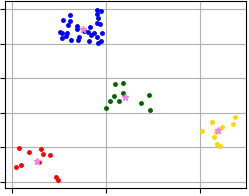pyclustering.cluster.xmeans.xmeans Class Reference

Class represents clustering algorithm X-Means. More...

## Public Member Functions

def __init__ (self, data, initial_centers=None, kmax=20, tolerance=0.025, criterion=splitting_type.BAYESIAN_INFORMATION_CRITERION, ccore=True)
Constructor of clustering algorithm X-Means. More...

def process (self)
Performs cluster analysis in line with rules of X-Means algorithm. More...

def get_clusters (self)
Returns list of allocated clusters, each cluster contains indexes of objects in list of data. More...

def get_centers (self)
Returns list of centers for allocated clusters. More...

def get_cluster_encoding (self)
Returns clustering result representation type that indicate how clusters are encoded. More...

## Detailed Description

Class represents clustering algorithm X-Means.

X-means clustering method starts with the assumption of having a minimum number of clusters, and then dynamically increases them. X-means uses specified splitting criterion to control the process of splitting clusters. Method K-Means++ can be used for calculation of initial centers.

CCORE implementation of the algorithm uses thread pool to parallelize the clustering process.

Here example how to perform cluster analysis using X-Means algorithm:

from pyclustering.cluster import cluster_visualizer
from pyclustering.cluster.xmeans import xmeans
from pyclustering.cluster.center_initializer import kmeans_plusplus_initializer
from pyclustering.samples.definitions import SIMPLE_SAMPLES
# Read sample 'simple3' from file.
# Prepare initial centers - amount of initial centers defines amount of clusters from which X-Means will
# start analysis.
amount_initial_centers = 2
initial_centers = kmeans_plusplus_initializer(sample, amount_initial_centers).initialize()
# Create instance of X-Means algorithm. The algorithm will start analysis from 2 clusters, the maximum
# number of clusters that can be allocated is 20.
xmeans_instance = xmeans(sample, initial_centers, 20)
xmeans_instance.process()
# Extract clustering results: clusters and their centers
clusters = xmeans_instance.get_clusters()
centers = xmeans_instance.get_centers()
# Visualize clustering results
visualizer = cluster_visualizer()
visualizer.append_clusters(clusters, sample)
visualizer.append_cluster(centers, None, marker='*', markersize=10)
visualizer.show()

Visualization of clustering results that were obtained using code above and where X-Means algorithm allocates four clusters.Fig. 1. X-Means clustering results (data 'Simple3').
center_initializer

Definition at line 78 of file xmeans.py.

## ◆ __init__()

 def pyclustering.cluster.xmeans.xmeans.__init__ ( self, data, initial_centers = `None`, kmax = `20`, tolerance = `0.025`, criterion = `splitting_type.BAYESIAN_INFORMATION_CRITERION`, ccore = `True` )

Constructor of clustering algorithm X-Means.

Parameters
 [in] data (list): Input data that is presented as list of points (objects), each point should be represented by list or tuple. [in] initial_centers (list): Initial coordinates of centers of clusters that are represented by list: [center1, center2, ...], if it is not specified then X-Means starts from the random center. [in] kmax (uint): Maximum number of clusters that can be allocated. [in] tolerance (double): Stop condition for each iteration: if maximum value of change of centers of clusters is less than tolerance than algorithm will stop processing. [in] criterion (splitting_type): Type of splitting creation. [in] ccore (bool): Defines should be CCORE (C++ pyclustering library) used instead of Python code or not.

Definition at line 126 of file xmeans.py.

## ◆ get_centers()

 def pyclustering.cluster.xmeans.xmeans.get_centers ( self )

Returns list of centers for allocated clusters.

Returns
(list) List of centers for allocated clusters.
process()
get_clusters()

Definition at line 202 of file xmeans.py.

## ◆ get_cluster_encoding()

 def pyclustering.cluster.xmeans.xmeans.get_cluster_encoding ( self )

Returns clustering result representation type that indicate how clusters are encoded.

Returns
(type_encoding) Clustering result representation.
get_clusters()

Definition at line 216 of file xmeans.py.

## ◆ get_clusters()

 def pyclustering.cluster.xmeans.xmeans.get_clusters ( self )

Returns list of allocated clusters, each cluster contains indexes of objects in list of data.

Returns
(list) List of allocated clusters.
process()
get_centers()

Definition at line 188 of file xmeans.py.

## ◆ process()

 def pyclustering.cluster.xmeans.xmeans.process ( self )

Performs cluster analysis in line with rules of X-Means algorithm.

Remarks
Results of clustering can be obtained using corresponding gets methods.